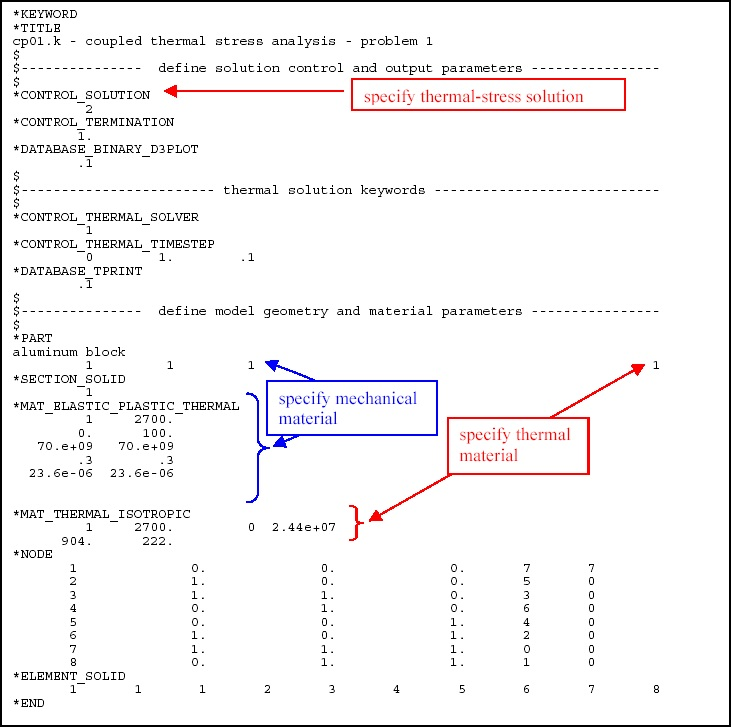# The Next Step

Home » Knowledge Base » Simple Examples » General » The Next Step

This chapter builds on the simple example presented in the previous chapter.

First, more detail is given about solving this problem using explicit analysis in section 4.1. Explicit analysis is well suited to dynamic simulations such as impact and crash analysis, but it can become prohibitively expensive to conduct long duration or static analyses. Static problems, such as sheet metal spring back after forming, are one application area for implicit analysis. Implicit analysis is presented in section 4.2. The difference between explicit and implicit is described. The problem is then presented as a heat transfer problem in section 4.3 and finally as a coupled thermal-stress problem in section 4.4.

## Explicit Analysis (problem ex01.k)

Explicit refers to the numerical method used to represent and solve the time derivatives in the momentum and energy equations. The following figure presents a graphical description of explicit time integration.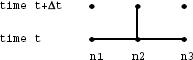The displacement of node n2 at time level t+ t is equal to known values of the displacement at nodes n1, n2, and n3 at time level t. A system of explicit algebraic equations are written for all the nodes in the mesh at time level t+ t. Each equation is solved in-turn for the unknown node point displacements. Explicit methods are computational fast but are conditionally stable. The time step, t, must be less than a critical value or computational errors will grow resulting in a bad solution. The time step must be less than the length of time it takes a signal traveling at the speed of sound in the material to traverse the distance between the node points. The critical time step for this problem can be calculated by: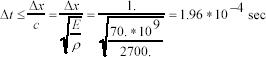To be safe, the default value used by LS-DYNA is 90% of this value or 1.77e-04 sec. Therefore, this problem requires 5,658 explicit time steps as compared with 10 implicit time steps (see section 4.2). Note that the time step and scale factor can be set using the keyword `*CONTROL_TIMESTEP`. The input file for the 1-element aluminum cube example problem, presented in Chapter 3, is duplicated below. The keyword `*TITLE` has been added for problem identification.

## Example 4-1 Aluminum cube deformation, explicit method (file: ex01.k)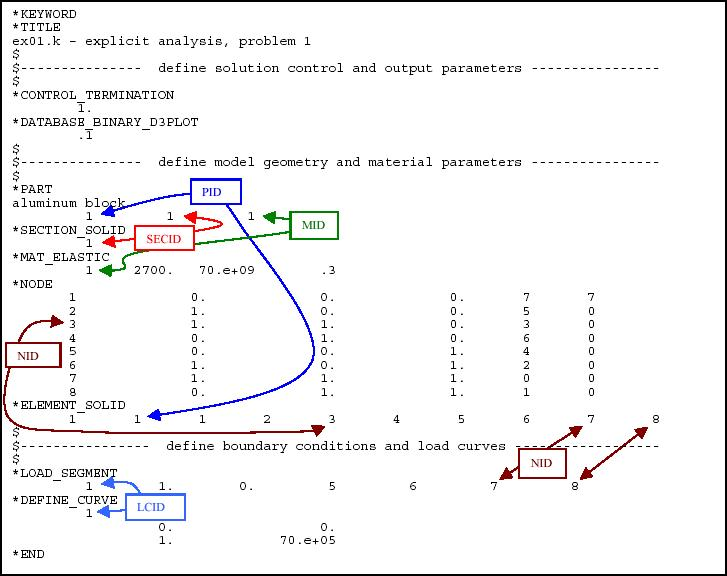## Implicit Analysis (problem im01.k)

Implicit refers to the numerical method used to represent and solve the time derivatives in the momentum and energy equations. The following figure presents a graphical description of implicit time integration.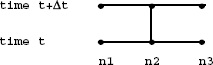The displacement of node n2 at time level t+ t is equal to known values of the displacement at nodes n1, n2, and n3 at time level t, and also the unknown displacements of nodes n1 and n3 at time level t+ t. This results in a system of simultaneous algebraic equations that are solved using matrix algebra (e.g., matrix inversion). The advantage of this approach is that it is unconditionally stable (i.e., there is no critical time step size). The disadvantage is the large numerically effort required to form, store, and invert the system of equations. Implicit simulations typically involve a relatively small number of computationally expensive time steps.

## Example 4-2 Aluminum cube deformation, implicit method (file: im01.k):

`    *KEYWORD      *TITLE      im01.k   implicit analysis   problem 1      \$      \$-------------------------- implicit solution keywords -------------------------      \$      *CONTROL_IMPLICIT_GENERAL              1        .1      \$--------------- define solution control and output parameters -----------------      \$      *CONTROL_TERMINATION              1.      *DATABASE_BINARY_D3PLOT             .1     \$      \$--------------- define model geometry and material parameters ----------------      \$      *PART      aluminum block               1         1         1      *SECTION_SOLID               1      *MAT_ELASTIC               1     2700.   70.e+09        .3      *NODE             1              0.              0.              0.       7       7             2              1.              0.              0.       5       0             3              1.              1.              0.       3       0             4              0.              1.              0.       6       0            5              0.              0.              1.       4       0            6              1.              0.              1.       2       0            7              1.              1.              1.       0       0            8              0.              1.              1.       1       0     *ELEMENT_SOLID             1       1       1       2       3       4       5       6       7       8     \$      \$---------------- define boundary conditions and load curves ------------------     \$      *LOAD_SEGMENT               1        1.        0.         5         6         7         8      *DEFINE_CURVE               1                        0.                  0.                       1.             70.e+05      *END`

## Heat Transfer Analysis (problem th01.k)

LS-DYNA can solve steady state and transient heat transfer problems. Steady state problems are solved in one step, while transient problems are solved using an implicit method. Our 1-element problem will now be re-defined as a transient heat transfer problem as shown below: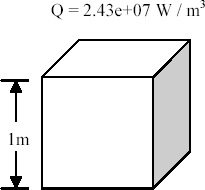Aluminum 1100-O density 2700 kg/m3 modulus of elasticity 70.0 e+09 Pa Poisson Ratio 0.3 coefficient of expansion 3.6e-06 m/m K heat capacity 900 J/kg K thermal conductivity 220 W/m K

We will solve for the temperature response of the cube as the result of internal heat generation, Q. All the surfaces of the cube are perfectly insulated. Therefore, all the heat generation goes into increasing the internal energy of the cube. The temperature response of the cube calculated by LSDYNA and the analytical solution are shown below.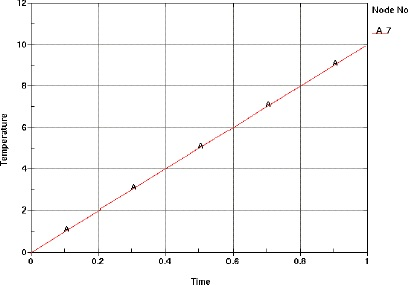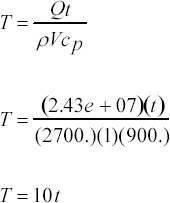The keyword input for this problem is shown below. Important things to note are:

• The default initial condition is T=0 for all nodes.
• The default thermal boundary condition is adiabatic (i.e. perfectly insulted) Therefore, no thermal boundary conditions need be specified for this problem.
• The `*CONTROL_SOLUTION` keyword is used to specify this problem as thermal only
• The entry on the `*PART` keyword points to the definition of thermal property data

## Coupled thermal-stress analysis (problem cp01.k)

In this problem, the cube is allowed to expand due to the temperature increase from internal heat generation. Keywords from the mechanical problem defined in section 4.1 and the thermal problem defined in section 4.3 are combined to define this thermal-stress problem. The keyword `*MAT_ELASTIC_PLASTIC_THERMAL` is used to define a material with a thermal coefficient of expansion. For this problem, alpha =23.6-06 m/m C. The aluminum blocks starts out at 0C (the default initial condition) and heats up 10C over the 1 second time interval (see section 1.3 above). The x displacement of node 7 versus temperature increase as calculated by LS-DYNA is shown in the figure below. The curve is not smooth due to numerical noise in the solution because we are only using 1 element. The analytical solution is shown below.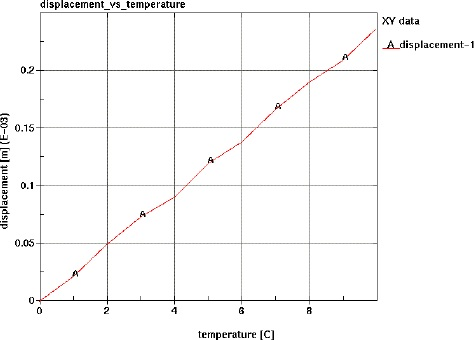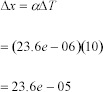The keyword input for this problem is shown below. Important things to note are:

• `*CONTROL_SOLUTION` is set to 2. This defines the problem as a coupled thermal stress analysis.
• Defining both mechanical and thermal properties.
• Using a mechanical constitutive model (`*MAT_ELASTIC_PLASTIC_THERMAL`) that allows entry of a thermal coefficient of expansion and mechanical properties that are a function of temperature.
• The mechanical and thermal time steps are independent. For this problem, we are using the default explicit mechanical time step calculated by the code and have specified a thermal time step of 0.1 sec. Therefore, many mechanical time steps will be taken for every thermal time step.

## Example 4-4 Aluminum cube coupled thermal-stress solution (file: cp01.k)